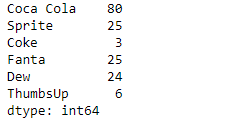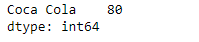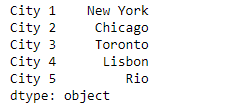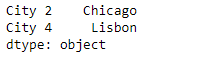# Python | Pandas Series.filter()

Pandas series is a One-dimensional ndarray with axis labels. The labels need not be unique but must be a hashable type. The object supports both integer- and label-based indexing and provides a host of methods for performing operations involving the index.

Pandas` Series.filter()` function returns subset rows or columns of dataframe according to labels in the specified index. Please note that this routine does not filter a dataframe on its contents. The filter is applied to the labels of the index.

Syntax: Series.filter(items=None, like=None, regex=None, axis=None)

Parameter :
items : List of axis to restrict to (must not all be present).
like : Keep axis where “arg in col == True”.
regex : Keep axis with re.search(regex, col) == True.
axis : The axis to filter on. By default this is the info axis, ‘index’ for Series, ‘columns’ for DataFrame.

Returns : same type as input object

Example #1: Use `Series.filter()` function to filter out some values in the given series object using a regular expressions.

 `# importing pandas as pd ` `import` `pandas as pd ` ` `  `# Creating the Series ` `sr ``=` `pd.Series([``80``, ``25``, ``3``, ``25``, ``24``, ``6``]) ` ` `  `# Create the Index ` `index_ ``=` `[``'Coca Cola'``, ``'Sprite'``, ``'Coke'``, ``'Fanta'``, ``'Dew'``, ``'ThumbsUp'``] ` ` `  `# set the index ` `sr.index ``=` `index_ ` ` `  `# Print the series ` `print``(sr) `

Output :Now we will use `Series.filter()` function to filter those values from the given series object whose index label name has a space in its name.

 `# filter values ` `result ``=` `sr.``filter``(regex ``=` `'. .'``) ` ` `  `# Print the result ` `print``(result) `

Output :As we can see in the output, the `Series.filter()` function has successfully returned the desired values from the given series object.

Example #2 : Use `Series.filter()` function to filter out some values in the given series object using a list of index labels.

 `# importing pandas as pd ` `import` `pandas as pd ` ` `  `# Creating the Series ` `sr ``=` `pd.Series([``'New York'``, ``'Chicago'``, ``'Toronto'``, ``'Lisbon'``, ``'Rio'``]) ` ` `  `# Create the Index ` `index_ ``=` `[``'City 1'``, ``'City 2'``, ``'City 3'``, ``'City 4'``, ``'City 5'``]  ` ` `  `# set the index ` `sr.index ``=` `index_ ` ` `  `# Print the series ` `print``(sr) `

Output :Now we will use `Series.filter()` function to filter the values corresponding to the passed index labels in the given series object.

 `# filter values ` `result ``=` `sr.``filter``(items ``=` `[``'City 2'``, ``'City 4'``]) ` ` `  `# Print the result ` `print``(result) `

Output :As we can see in the output, the `Series.filter()` function has successfully returned the desired values from the given series object.

My Personal Notes arrow_drop_upCheck out this Author's contributed articles.

If you like GeeksforGeeks and would like to contribute, you can also write an article using contribute.geeksforgeeks.org or mail your article to contribute@geeksforgeeks.org. See your article appearing on the GeeksforGeeks main page and help other Geeks.

Please Improve this article if you find anything incorrect by clicking on the "Improve Article" button below.

Article Tags :

Be the First to upvote.

Please write to us at contribute@geeksforgeeks.org to report any issue with the above content.